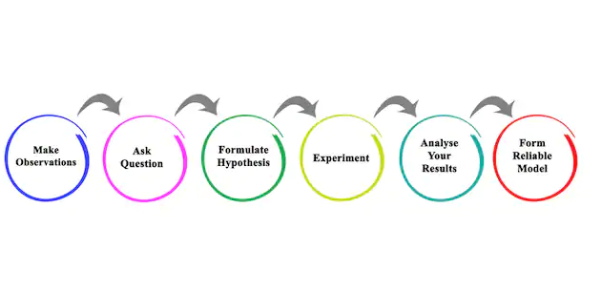# Scientific Method MCQ Quiz!

10 Questions | Attempts: 1994Settings.

• 1.
Which of the following is true?
• A.

A theory can turn into a law

• B.

Several laws can result in a theory

• C.

A law is the top level of the scientific method

• D.

A theory is just a hypothesis

• 2.
A well-tested scientific theory is a fact.
• A.

True

• B.

False

• 3.
Which of these is not a scientific theory?
• A.

Plate tectonics (the continents are in motion)

• B.

Cell theory (all living things are made of cells)

• C.

Atomic theory (everything is made of atoms)

• D.

Those are all scientific theories

• 4.
Which order is correct for the Scientific Method?
• A.

Observation, Hypothesis, Testing, Analysis, Conclusion

• B.

Hypothesis, Observation, Conclusion, Testing, Analysis

• C.

Hypothesis, Testing, Analysis, Conclusion, Observation

• D.

Observation, Hypothesis, Testing, Analysis, Conclusion

• 5.
A scientific theory is an explanation for multiple scientific laws.
• A.

True

• B.

False

• 6.
A law explains multiple theories.
• A.

True

• B.

False

• 7.
A scientific theory:
• A.

Like a hypothesis it is a "guess".

• B.

May progress to a law

• C.

Has to be proven to be true

• D.

Allows scientists to make and test predictions

• 8.
Science proves things.
• A.

True: If it doesn't prove something is true, it's not a theory

• B.

False: You want proofs, study Geometry!

• 9.
Laws can be very complex, but theories are simple concepts.
• A.

True

• B.

False

• 10.
How does the Scientific Method work?
• A.

You learn enough about something to make an educated guess, then try to show it is wrong.

• B.

A repeatable, well-tested rule learned from multiple experiments can become a law.

• C.

Scientific Laws simply state a fact: an explanation for how they work is not always known (yet).

• D.

Why a law (usually several laws) works is called a Scientific Theory

• E.

All of the above are part of the Scientific Method

## Related TopicsBack to top
×

Wait!
Here's an interesting quiz for you.